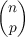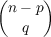#### Vol. 26, No. 2, 1968

 Download this articleFor screen For printingRecent Issues Vol. 325: 1 Vol. 324: 1  2 Vol. 323: 1  2 Vol. 322: 1  2 Vol. 321: 1  2 Vol. 320: 1  2 Vol. 319: 1  2 Vol. 318: 1  2Online Archive Volume: Issue:The Journal Subscriptions Editorial Board Officers Contacts Submission Guidelines Submission Form Policies for Authors ISSN: 1945-5844 (e-only) ISSN: 0030-8730 (print) Special Issues Author Index To Appear Other MSP Journals
Bounded series and Hausdorff matrices for absolutely convergent sequences

### Philip C. Tonne

Vol. 26 (1968), No. 2, 415–420
##### Abstract

If f is a function from [0,1] to the complex plane and c is a complex sequence, then the Hausdorff matrix H(c) for c and a sequence L(f,c) are defined:

 H(c)np =∑ q=0n−p(−1)qcp+q L(f,c)n = ∑ p=0nH(c) npf(p∕n).
This paper consists of the following theorem and two converses to it.

Theorem 1. If A is a complex sequence and p=0Ap is bounded (there is a number B such that if n is a nonnegative integer then | p=0nAp| < B), f is a function from [0,1] to the complex plane such that if 0 x < 1 then f(x) = p=0Apxp, and c is an absolutely convergent sequence ( p=0|cp+1 cp| converges), then L(f,c) converges. Furthermore, if c has limit d, L(f,c) has limit p=0Ap(cp d) + f(1)d.

Primary: 30.30
##### Milestones